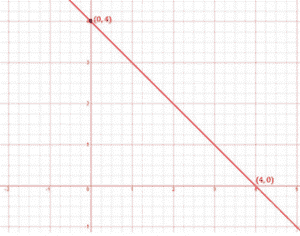Newbie

# Draw the graph of each of the following linear equations in two variables: (i) x+y = 4 Q.1(1)

• 1

How i solve the ncert class 9th question of chapter linear equations in two variables of exercise 4.3 question number 1(1). Please give me the simplest and easiest solution of this question  ,also give me the best solution of this question Draw the graph of each of the following linear equations in two variables: (i) x+y = 4

Share

1. Solution:

To draw a graph of linear equations in two variables, let us find out the points to plot.

To find out the points, we have to find the values which x and y can have, satisfying the equation.

Here,

x+y = 4

Substituting the values for x,

When x = 0,

x+y = 4

0+y = 4

y = 4

When x = 4,

x+y = 4

4+y = 4

y = 4–4

y = 0

 x y 0 4 4 0

The points to be plotted are (0, 4) and (4,0)• 0